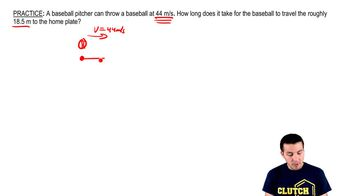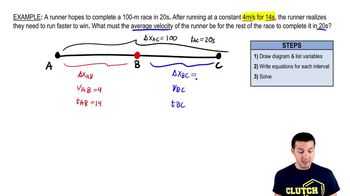Start typing, then use the up and down arrows to select an option from the list.
2:32 minutes
Problem 2b
Textbook Question

# A car is stopped at a traffic light. It then travels along a straight road such that its distance from the light is given by x(t) = bt2 − ct3, where b = 2.40 m/s2 and c = 0.120 m/s3. (a) Calculate the average velocity of the car for the time interval t = 0 to t = 10.0 s.Verified Solution
This video solution was recommended by our tutors as helpful for the problem above.
225views